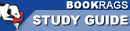Study Guide

# Archimedes and the Door of Science - Chapter 8, The Measurement of a Circle Summary & Analysis

This Study Guide consists of approximately 20 pages of chapter summaries, quotes, character analysis, themes, and more - everything you need to sharpen your knowledge of Archimedes and the Door of Science.
 This section contains 211 words (approx. 1 page at 400 words per page) View a FREE sample## Chapter 8, The Measurement of a Circle Summary and Analysis

Multiplying two times the radius of a circle time pi, 3.14159, gets you the circumference of a circle. Pi times that radius squared renders the area of the circle. But Archimedes figured out how to find the area of a circle without the formula. Until Archimedes, people found out the approximate area of a circle by drawing the largest square they could within a circle and then the smallest square outside of it. The area of the circle was somewhat in between the area of the two squares. It would also help with the circle.

Archimedes enclosed his circle with polygons, adding more sides until he reached ninety-six. The circumference he found with his polygons was always less than 22/7ths multiplied times the diameter and always more than 223/71sts the diameters. He...

(read more from the Chapter 8, The Measurement of a Circle Summary)

 This section contains 211 words (approx. 1 page at 400 words per page) View a FREE sample